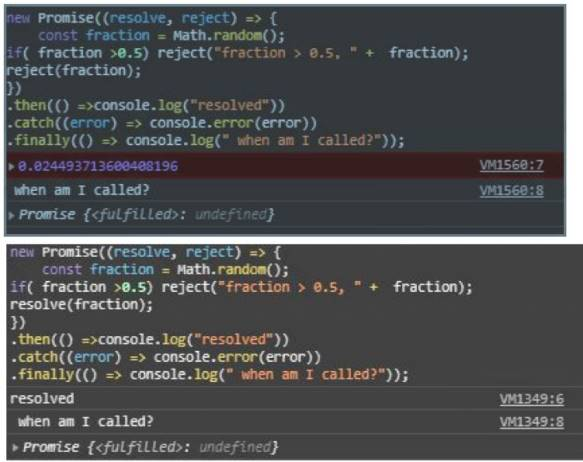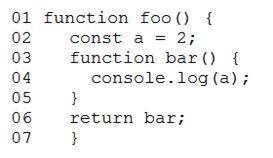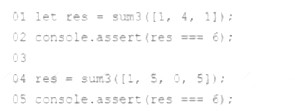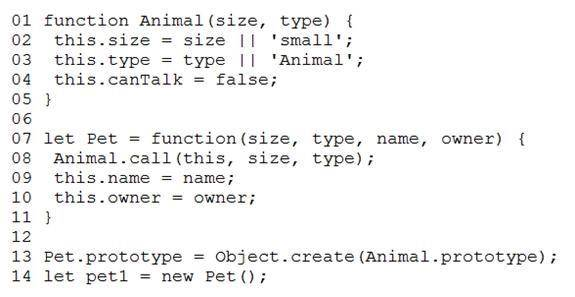Your success in Salesforce JavaScript-Developer-I is our sole target and we develop all our JavaScript-Developer-I braindumps in a way that facilitates the attainment of this target. Not only is our JavaScript-Developer-I study material the best you can find, it is also the most detailed and the most updated. JavaScript-Developer-I Practice Exams for Salesforce JavaScript-Developer-I are written to the highest standards of technical accuracy.

Online Salesforce JavaScript-Developer-I free dumps demo Below:

NEW QUESTION 1
Refer to the code below:
new Promise((resolve, reject) => { const fraction = Math.random();
if( fraction >0.5) reject("fraction > 0.5, " + fraction); resolve(fraction);
})
.then(() =>console.log("resolved"))
.catch((error) => console.error(error))
.finally(() => console.log(" when am I called?"));When does Promise.finally on line 08 get called?

• A. When rejected
• B. When resolved and settled
• C. WHen resolved
• D. When resolved or rejected

NEW QUESTION 2
A developer wants to define a function log to be used a few times on a single-file JavaScript script.
01 // Line 1 replacement
03 }
Which two options can correctly replace line 01 and declare the function for use? Choose 2 answers

• C. const log = (logInput) => {
• D. function log = (logInput) {

NEW QUESTION 3
Given two expressions var1 and var2. What are two valid ways to return the logical AND of the two expressions and ensure it is data typeBoolean ?

• A. Boolean(var1 && var2)
• B. var1 && var2
• C. var1.toBoolean() && var2toBoolean()
• D. Boolean(var1) && Boolean(var2)

NEW QUESTION 4
Which option is true about the strict mode in imported modules?

• A. Add the statement use non-strict, before any other statements in the module to enable not-strict mode.
• B. You can only reference notStrict() functions from the imported module.
• C. Imported modules are in strict mode whether you declare them as such or not.
• D. Add the statement use strict =false; before any other statements in the module to enable not- strict mode.

NEW QUESTION 5
Why would a developer specify a package.jason as a developed forge instead of a dependency ?

• A. It is required by the application in production.
• B. It is only needed for local development and testing.
• C. Other requiredpackages depend on it for development.
• D. It should be bundled when the package is published.

NEW QUESTION 6
A developer wrote a fizzbuzz function that when passed in a number, returns the following:
‘Fizz’ if the number is divisible by 3. ‘Buzz’ if the number is divisible by 5.
‘Fizzbuzz’ if the number is divisible by both 3 and 5. Emptystring if the number is divisible by neither 3 or 5.
Which two test cases will properly test scenarios for the fizzbuzz function? Choose 2 answers

• A. let res = fizzbuzz(5); console.assert ( res === ‘ ’ );
• B. let res = fizzbuzz(15);console.assert ( res ===‘ fizzbuzz ’ )
• C. let res = fizzbuzz(Infinity); console.assert ( res === ‘ ’ )
• D. let res = fizzbuzz(3); console.assert ( res === ‘ buzz ’ )

NEW QUESTION 7
Refer to the following code that performs a basic mathematical operation on a provided input:
function calculate(num) { Return (num +10) / 3;
}
How should line 02 be written to ensure thatx evaluates to 6 in the line below? Let x = calculate (8);

• A. Return Number((num +10) /3 );
• B. Return (Number (num +10 ) / 3;
• C. Return Integer(num +10) /3;
• D. Return Number(num + 10) / 3;

NEW QUESTION 8
Refer to HTML below:
<div id =”main”>
<div id = “ card-00”>This card is smaller.</div>
<div id = “card-01”>The width and height of this card is determined by its contents.</div>
</div>
Which expression outputs the screen width of the element with the ID card-01?

• A. document.getElementById(‘ card-01 ’).getBoundingClientRest().width
• B. document.getElementById(‘ card-01 ’).style.width
• C. document.getElementById(‘ card-01 ’).width
• D. document.getElementById(‘ card-01 ’).innerHTML.lenght*e

NEW QUESTION 9
Refer to the code below:• A. Inner function’s scope
• B. Hoisting
• C. Outer function’s scope
• D. Prototype chain

NEW QUESTION 10
A team that works on a big project uses npm to deal with projects dependencies. A developer added a dependency does not get downloaded when they executenpm install.
Which two reasons could be possible explanations for this?

• A. The developer missed the option --add when adding the dependency.
• B. The developer added the dependency as a dev dependency, and NODE_ENVIs set to production.
• C. The developer missed the option --save when adding the dependency.
• D. The developer added the dependency as a dev dependency, and NODE_ENV is set to production.

NEW QUESTION 11
What are two unique features of functions defined with a fat arrow as compared to normal function definition?

• A. The function generated its own this making ituseful for separating the function’s scope from its enclosing scope.
• B. The function receives an argument that is always in scope, called parentThis, which is the enclosing lexical scop
• C. If the function has a single expression in the function body, the expression will be evaluated and implicit returned.
• D. The function uses the this from the enclosing scope.

NEW QUESTION 12
A developer has code that calculates a restaurant bill, but generates incorrectanswers while testing the code:
function calculateBill ( items ) { let total = 0;
total += findSubTotal(items); total += addTax(total);
}
Which option allows the developer to step into each function execution within calculateBill?

• A. Using the debugger command on line 05.
• B. Using the debugger command on line 03
• C. Calling the console.trace (total) method on line 03.
• D. Wrapping findSubtotal in a console.log() method.

NEW QUESTION 13
Refer to the code below: FunctionPerson(firstName, lastName, eyecolor) { this.firstName =firstName;
this.lastName = lastName;
this.eyeColor = eyeColor;
}
Person.job = ‘Developer’;
const myFather = new Person(‘John’, ‘Doe’); console.log(myFather.job);
What is the output after the codeexecutes?

• A. ReferenceError: eyeColor is not defined
• B. ReferenceError: assignment to undeclared variable “Person”
• C. Developer
• D. Undefined

NEW QUESTION 14
A developer wrote the following codeto test a sum3 function that takes in an array of numbers and returns the sum of the first three numbers in the array, and the test passes.
A different developer made changes to the behavior of sum3 to instead sum only the first two numbers present in thearray.Which two results occur when running this test on the updated sum3 function? Choose 2 answers

• A. The line 05 assertion passes.
• B. The line 02 assertion passes.
• C. The line 02 assertion fails.
• D. The line 05 assertion fails.

NEW QUESTION 15
Given the following code: Let x =(‘15’ + 10)*2;
What is the value of a?

• A. 3020
• B. 1520
• C. 50
• D. 35

NEW QUESTION 16
Refer to the code:Given the code above, which three properties are set pet1? Choose 3answers:

• A. Name
• B. canTalk
• C. Type
• D. Owner
• E. Size